We often use gradients of some kind in shaders, but there are cases where we’re limited to less shades of colors than we want to express. One common technique to fake having many different colors with only a few is dithering. In this tutorial I explain how to dither between two colors based on a given ratio, but it’s also possible to use dithering for more shades of color with more complex algorithms.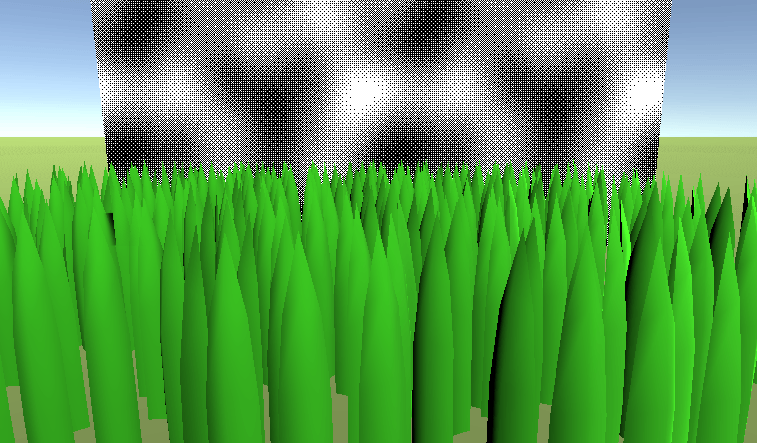## Simple Dithering

For this first version we’re taking the red channel of the input texture as the ratio between the two colors. For the pattern how to combine them we use a “bayer dithering” pattern, it’s optimized to have as much difference between the neighboring pixels in the pattern. As the base for this shader I used the result of the unlit shader with texture access.

Getting access to the base color we want to dither is already done with with this texture sample, but we don’t know how to read from the dither pattern texture. Unless you use fancy mapping techniques like Return of Obra Dinn did, the most straightforward approach here is to use screenspace UV coordinates. I explain how to get the basic screenspace coordinates in this tutorial. One thing that’s pretty special about dithering is that we don’t care about how big the dither texture is or how often it repeats on the screen. The only thing we care about is that one texture pixel maps to one screen pixel to use it exactly as intended. To archieve that we first multiply the sceenspace UVs by the screen size itself, creating a UV set that increases by 1 for every pixel. Then we divide that UV by the amount of pixels of the dither texture, creating a texture that goes from 0 to 1 every “dither texture size” pixels, always sampling the middle of the pixels.

When doing those calculations we can easily get the screen size from the x and y components of the builtin `_ScreenParams` variable. To get the size of the dither pattern we add a new variable to the shader that has the same name as the texture we want to know the size of, but with `_TexelSize` to the end of it’s name. Then instead of dividing by the size of the texture (the z and w components of this vector) we can also multiply with one divided by the size, this value is already saved in the x and y components of this vector. We do this because a multiplication is usually faster than a division.

Here are the 4x4 and 8x8 versions of the dither texture I used: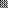It’s important to disable compression completely in unity, otherwise it will mess with your textures and it will look bad (the textures are so tiny that compression wouldn’t make much of a difference anyways). Which texture you use doesn’t matter that much, the 8x8 texture gives you similar results in small areas and leads to less banding with slowly changing values, so if you’re not sure use the bigger one.

``````//Shader Property
_DitherPattern ("Dithering Pattern", 2D) = "white" {}
``````
``````//Shader Variables

//The dithering pattern
sampler2D _DitherPattern;
float4 _DitherPattern_TexelSize;
``````
``````//the data that's used to generate fragments and can be read by the fragment shader
struct v2f{
float4 position : SV_POSITION;
float2 uv : TEXCOORD0;
float4 screenPosition : TEXCOORD1;
};
``````
``````//test fragment shader
fixed4 frag(v2f i) : SV_TARGET{
float2 screenPos = i.screenPosition.xy / i.screenPosition.w;
float2 ditherCoordinate = screenPos * _ScreenParams.xy * _DitherPattern_TexelSize.xy;
float ditherValue = tex2D(_DitherPattern, ditherCoordinate).r;
return ditherValue;
}
``````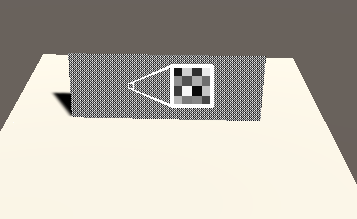With this value in hand we can already compare it to the density of the dithering and render the result. For this case the `step` function is ideal, we can pipe in the dither value and the value of our texture to get a 0 or 1 binary result that’ll represent the value of the texture value by regulating the density of the pixels.

``````//the fragment shader
fixed4 frag(v2f i) : SV_TARGET{
//texture value the dithering is based on
float texColor = tex2D(_MainTex, i.uv).r;

//value from the dither pattern
float2 screenPos = i.screenPosition.xy / i.screenPosition.w;
float2 ditherCoordinate = screenPos * _ScreenParams.xy * _DitherPattern_TexelSize.xy;
float ditherValue = tex2D(_DitherPattern, ditherCoordinate).r;

//combine dither pattern with texture value to get final result
float col = step(ditherValue, texColor);
return col;
}
``````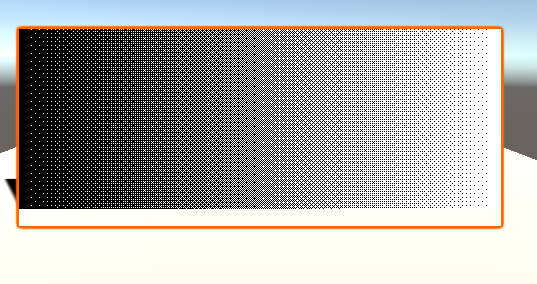If you want to make the dither colors anything but black/white you can use a linear interpolation with the value we just used as a color as the interpolation parameter.

``````//Shader Properties
_Color1 ("Dither Color 1", Color) = (0, 0, 0, 1)
_Color2 ("Dither Color 2", Color) = (1, 1, 1, 1)
``````
``````//Shader variables
float4 _Color1;
float4 _Color2;
``````
``````//the fragment shader
fixed4 frag(v2f i) : SV_TARGET{
//texture value the dithering is based on
float texColor = tex2D(_MainTex, i.uv).r;

//value from the dither pattern
float2 screenPos = i.screenPosition.xy / i.screenPosition.w;
float2 ditherCoordinate = screenPos * _ScreenParams.xy * _DitherPattern_TexelSize.xy;
float ditherValue = tex2D(_DitherPattern, ditherCoordinate).r;

//combine dither pattern with texture value to get final result
float ditheredValue = step(ditherValue, texColor);
float4 col = lerp(_Color1, _Color2, ditheredValue);
return col;
}
``````When we want to do the same in a surface shader the steps we have to take are a bit easier since we don’t have to write our own vetex shader to get access to the screenspace coordinates. Instead we just just have to add a variable called `ScreenPos` to the input struct. If you want to do this but don’t know yet how surface shaders work like, read the tutorial about them here.

``````//input struct which is automatically filled by unity
struct Input {
float2 uv_MainTex;
float4 screenPos;
};

//the surface shader function which sets parameters the lighting function then uses
void surf (Input i, inout SurfaceOutputStandard o) {
//texture value the dithering is based on
float texColor = tex2D(_MainTex, i.uv_MainTex).r;

//value from the dither pattern
float2 screenPos = i.screenPos.xy / i.screenPos.w;
float2 ditherCoordinate = screenPos * _ScreenParams.xy * _DitherPattern_TexelSize.xy;
float ditherValue = tex2D(_DitherPattern, ditherCoordinate).r;

//combine dither pattern with texture value to get final result
float ditheredValue = step(ditherValue, texColor);
o.Albedo = lerp(_Color1, _Color2, ditheredValue);
}
``````

If you do this you might notice that the end result on your screen has a gradient based on the lighting. If you don’t want that you can also write your own lighting function and implement the dithering after the lighting was calculated, but I’m not going into that in this tutorial.

I explained how to make your materials look transparent in an earlier tutorial, but when we use this our material becomes much more expensive to render because it becomes more important in which order objects are rendered since we can’t use the Z-buffer anymore (it’s not critical to understand what this means) and all objects at a position have to be rendered because the colors might mix. A solution to this problem is that we can discard pixels completely with the `clip` function. The main draw of this function is that it can only completely draw or discard a pixel, no inbetween values, this is where dithering comes in, but by drawing some of the pixels and discarding the rest with a dither pattern the result looks again like a semitransparent surface. In this example I’m going to show how to convert the surface shader variant into a shader that fades out when it comes close to the camera, but it works just as well for the unlit variant.

The clip function discards a pixel when it’s passed a value that’s lower than 0 and does nothing if the argument is 0 or higher. We can use this to do dithered transparency without even using the step function. By subtracting the dither pattern from the value we want to encode as a dithered pattern we get values that are lower than 0 in the correct relation to values that are not.

If you use the alpha channel instead of the red channel for discarding pixels here you can do normal transparency like this without having to pay the full rendering cost that comes with it. (There are other disadvantages, but it’s worth a shot if that’s whats killing your performance. Especially in particles where you can get a lot of overdraw otherwise this can be useful)

``````//the surface shader function which sets parameters the lighting function then uses
void surf (Input i, inout SurfaceOutputStandard o) {
//read texture and write it to diffuse color
float4 texColor = tex2D(_MainTex, i.uv_MainTex);
o.Albedo = texColor.rgb;

//value from the dither pattern
float2 screenPos = i.screenPos.xy / i.screenPos.w;
float2 ditherCoordinate = screenPos * _ScreenParams.xy * _DitherPattern_TexelSize.xy;
float ditherValue = tex2D(_DitherPattern, ditherCoordinate).r;

clip(texColor.r - ditherValue);
}
``````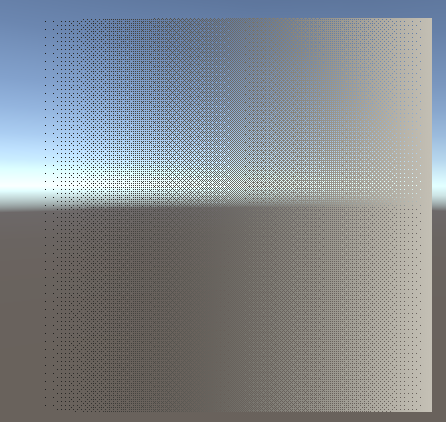We can already get the approximate distance to the camera by taking the 4th component of the screen position variable. After we have that we can then remap it to be 0 at the closest distance where surface is completely hidden and 1 at the furthest distance where the surface is completely visible. This operation is like the opposite of a linear interpolation and we can do it by first ensuring the zero is correct by subtracting the minimum fade distance from the distance and then dividing the result by the different between the maximum and minimum fade distance to move the point that was equal to the maximum before to 1.

``````//the surface shader function which sets parameters the lighting function then uses
void surf (Input i, inout SurfaceOutputStandard o) {
//read texture and write it to diffuse color
float3 texColor = tex2D(_MainTex, i.uv_MainTex);
o.Albedo = texColor.rgb;

//value from the dither pattern
float2 screenPos = i.screenPos.xy / i.screenPos.w;
float2 ditherCoordinate = screenPos * _ScreenParams.xy * _DitherPattern_TexelSize.xy;
float ditherValue = tex2D(_DitherPattern, ditherCoordinate).r;

//get relative distance from the camera
float relDistance = i.screenPos.w;
relDistance = relDistance - _MinDistance;
relDistance = relDistance / (_MaxDistance - _MinDistance);
clip(relDistance - ditherValue);
}
``````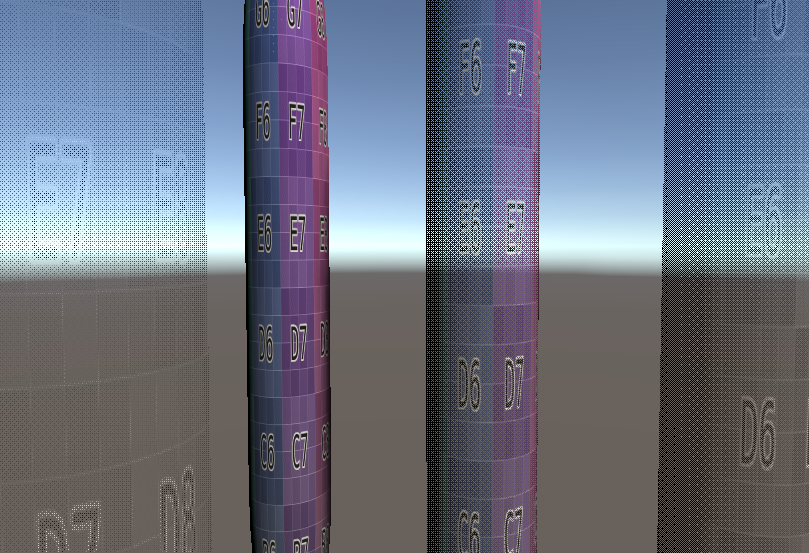## Source

### Unlit Binary Dither

``````Shader "Tutorial/042_Dithering/Basic"{
//show values to edit in inspector
Properties{
_MainTex ("Texture", 2D) = "white" {}
_DitherPattern ("Dithering Pattern", 2D) = "white" {}
_Color1 ("Dither Color 1", Color) = (0, 0, 0, 1)
_Color2 ("Dither Color 2", Color) = (1, 1, 1, 1)
}

//the material is completely non-transparent and is rendered at the same time as the other opaque geometry
Tags{ "RenderType"="Opaque" "Queue"="Geometry"}

Pass{
CGPROGRAM

#include "UnityCG.cginc"

#pragma vertex vert
#pragma fragment frag

//texture and transforms of the texture
sampler2D _MainTex;
float4 _MainTex_ST;

//The dithering pattern
sampler2D _DitherPattern;
float4 _DitherPattern_TexelSize;

//Dither colors
float4 _Color1;
float4 _Color2;

//the object data that's put into the vertex shader
struct appdata{
float4 vertex : POSITION;
float2 uv : TEXCOORD0;
};

//the data that's used to generate fragments and can be read by the fragment shader
struct v2f{
float4 position : SV_POSITION;
float2 uv : TEXCOORD0;
float4 screenPosition : TEXCOORD1;
};

v2f vert(appdata v){
v2f o;
//convert the vertex positions from object space to clip space so they can be rendered
o.position = UnityObjectToClipPos(v.vertex);
o.uv = TRANSFORM_TEX(v.uv, _MainTex);
o.screenPosition = ComputeScreenPos(o.position);
return o;
}

fixed4 frag(v2f i) : SV_TARGET{
//texture value the dithering is based on
float texColor = tex2D(_MainTex, i.uv).r;

//value from the dither pattern
float2 screenPos = i.screenPosition.xy / i.screenPosition.w;
float2 ditherCoordinate = screenPos * _ScreenParams.xy * _DitherPattern_TexelSize.xy;
float ditherValue = tex2D(_DitherPattern, ditherCoordinate).r;

//combine dither pattern with texture value to get final result
float ditheredValue = step(ditherValue, texColor);
float4 col = lerp(_Color1, _Color2, ditheredValue);
return col;
}

ENDCG
}
}

Fallback "Standard"
}
``````

``````Shader "Tutorial/042_Dithering/DistanceFade"{
//show values to edit in inspector
Properties{
_MainTex ("Texture", 2D) = "white" {}
_DitherPattern ("Dithering Pattern", 2D) = "white" {}
_MinDistance ("Minimum Fade Distance", Float) = 0
_MaxDistance ("Maximum Fade Distance", Float) = 1
}

//the material is completely non-transparent and is rendered at the same time as the other opaque geometry
Tags{ "RenderType"="Opaque" "Queue"="Geometry"}

CGPROGRAM

//the shader is a surface shader, meaning that it will be extended by unity in the background to have fancy lighting and other features
//our surface shader function is called surf and we use the default PBR lighting model
#pragma surface surf Standard
#pragma target 3.0

//texture and transforms of the texture
sampler2D _MainTex;

//The dithering pattern
sampler2D _DitherPattern;
float4 _DitherPattern_TexelSize;

//remapping of distance
float _MinDistance;
float _MaxDistance;

//input struct which is automatically filled by unity
struct Input {
float2 uv_MainTex;
float4 screenPos;
};

//the surface shader function which sets parameters the lighting function then uses
void surf (Input i, inout SurfaceOutputStandard o) {
//read texture and write it to diffuse color
float3 texColor = tex2D(_MainTex, i.uv_MainTex);
o.Albedo = texColor.rgb;

//value from the dither pattern
float2 screenPos = i.screenPos.xy / i.screenPos.w;
float2 ditherCoordinate = screenPos * _ScreenParams.xy * _DitherPattern_TexelSize.xy;
float ditherValue = tex2D(_DitherPattern, ditherCoordinate).r;

//get relative distance from the camera
float relDistance = i.screenPos.w;
relDistance = relDistance - _MinDistance;
relDistance = relDistance / (_MaxDistance - _MinDistance);